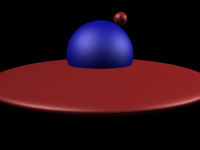#### CS 6620 - Fall 2017 - Ray Tracing for Graphics

Requirements

XML Scene Format

Our XML scene has some additional descriptions for materials and lights.```<xml>
<scene>
<!-- Objects -->
<object type="sphere" name="sphere1" material="mtl1">
<scale x="25" y="25" z="3"/>
<translate x="0" y="50" z="0"/>
</object>
<object type="sphere" name="sphere2" material="mtl2">
<scale value="8.0"/>
<rotate angle="30" y="1"/>
<translate x="0" y="50" z="5.1"/>
<object type="sphere" name="sphere3" material="mtl1">
<scale value="0.2"/>
<translate x="0" y="0" z="1.2"/>
</object>
</object>

<!-- Materials -->
<material type="blinn" name="mtl1">
<diffuse  r="0.8" g="0.2" b="0.2"/>
<specular r="1.0" g="1.0" b="1.0" value="0.7"/>
<glossiness value="20"/>
</material>
<material type="blinn" name="mtl2">
<diffuse  r="0.1" g="0.1" b="0.9"/>
<specular r="0.9" g="0.9" b="1.0" value="0.8"/>
<glossiness value="10"/>
</material>

<!-- Lights -->
<light type="ambient" name="ambientLight">
<intensity value="0.1"/>
</light>
<light type="direct" name="directionalLight">
<intensity value="0.5"/>
<direction x="1" y="0.5" z="-1"/>
</light>
<light type="point" name="pointLight">
<intensity value="0.5"/>
<position x="140" y="40" z="70"/>
</light>
</scene>

<camera>
<position x="0" y="0" z="10"/>
<target x="0" y="50" z="0"/>
<up x="0" y="0" z="1"/>
<fov value="40"/>
<width value="800"/>
<height value="600"/>
</camera>
</xml>
```

Source Code

The following source code files are provided to help you with this and upcoming projects. You are not required to use them, but it is highly recommened that you use them and refrain from modifying them. Some of these files include additional code as compared to the same files in the previous project, so make sure to download them all again.

• scene.h: Includes base classes for scene management.
• cyColor.h: Color classes from cyCodeBase, used by the scene.h file.
• xmlload.cpp: Includes the LoadScene() function that parses a given scene XML file using the TinyXML library.
• materials.h: Includes the description of the MtlBlinn class used by the xmlload.cpp file.
• lights.h: Includes the descriptions of the light classes used by the xmlload.cpp file.
• objects.h: Includes the description of the Sphere class used by the xmlload.cpp file.
• viewport.cpp: OpenGL based preview and user interface launched by calling the ShowViewport() function. It requires GLUT or FreeGLUT.

Optional Feature Suggestions

• Implement an additional material for Phong shading model and use "phong" as the material "type" in the scene definition.

Student Project Pages

_